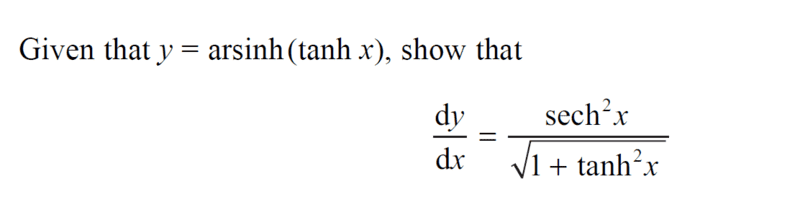# Comparing Hyperbolic and Cartesian Trig Properties

• chwala

#### chwala

Gold Member
Homework Statement
See attached.
Relevant Equations
hyperbolic trig. propertiesI came across this question; i noted that the hyperbolic trigonometry properties are somewhat similar to what i may call cartesian trigonometry properties...

My approach on this;

##\tanh x = \sinh y##

...just follows from

##y=\sin^{-1}(\tan x)##

##\tan x = \sin y##

Therefore continuing with our problem;

##\sech^{2}x= \cosh y \dfrac{dy}{dx}##

##⇒\dfrac{dy}{dx}= \dfrac{\sech^{2}x}{\cosh y}##

We know that;

##\cosh^2 y - \sinh^2y =1##

Therefore,

##\dfrac{dy}{dx}= \dfrac{\sech^{2}x}{\sqrt{1+\sinh^2y}}##

which gives us;

##\dfrac{dy}{dx}= \dfrac{\sech^{2}x}{\sqrt{1+\tanh^2x}}##

would appreciate insight or any other approach...

Last edited:
Why don't you simply use the chain rule?

•chwala